Download PDF文章快速检索 高级检索

1. 重庆邮电大学 计算机学院, 重庆 400065;
2. 重庆邮电大学 数理学院, 重庆 400065

Hybrid pruning algorithm for the neural network based on significance analysis
PU Xingcheng1,2, LIN Yanqin1
1. Department of Computer Science, Chongqing University of Post&Telecommunications, Chongqing 400065, China ;
2. Department of Mathematics&Physics, Chongqing University of Post&Telecommunications, Chongqing 400065, China
Abstract: This paper puts forward a kind of hybrid pruning algorithm for considering the problem of neural network structure design. Firstly, the algorithm uses the different advantages of cooperative co-evolutionary genetic algorithm and back propagation algorithm to optimize the structure and weights of neural networks. Secondly, by calculating the significance of the hidden layer neurons, it prunes the network that is not significant, further simplifying the structure of the network without reducing the generalization ability of the model. Finally, the proposed hybrid pruning algorithm is used to forecast the stock market. The simulations showed that the improved algorithm has better generalization ability and higher fitting precision than other optimization algorithms.
Key words: significance analysis     neural network     cooperative co-evolutionary genetic algorithms     pruning algorithm     stock market

1 混合修剪算法

1.1 种群分割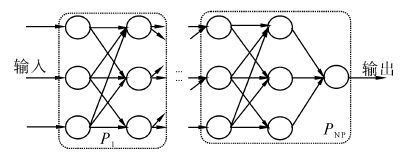图 1 种群分割图 Fig. 1 Population segmentation
1.2 决策变量编码

1.2.1 结构编码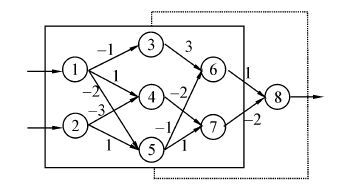图 2 神经网络编码图 Fig. 2 Neural network coding

 ${S_1} = \left[ {\begin{array}{*{20}{c}} \times & \times & \times & \times & \times & \times & \times \\ \times & \times & \times & \times & \times & \times & \times \\ 1&0& \times & \times & \times & \times & \times \\ 1&1& \times & \times & \times & \times & \times \\ 1&1& \times & \times & \times & \times & \times \\ \times & \times &1&0&1& \times & \times \\ \times & \times &0&1&1& \times & \times \end{array}} \right]$

 ${S_2} = \left[ {\begin{array}{*{20}{c}} \times & \times & \times & \times & \times & \times \\ \times & \times & \times & \times & \times & \times \\ \times & \times & \times & \times & \times & \times \\ 1&0&1& \times & \times & \times \\ 0&1&1& \times & \times & \times \\ \times & \times & \times &1&1& \times \end{array}} \right]$

1.2.2 权值编码

 $\begin{array}{l} {S_1} \to - 1,1, - 3, - 2,1,3, - 1, - 2,1,\\ {S_2} \to 3, - 1, - 2,1,1, - 2 \end{array}$
1.3 遗传操作

1.3.1 交叉操作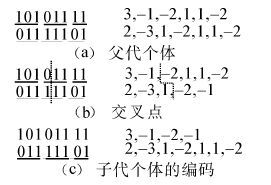图 3 交叉操作图 Fig. 3 Crossover operation chart

1.3.2 变异操作

1.4 基于显著性分析的神经网络结构优化

 $\Delta {E_{{\rm{av}}}}{\rm{ = }}{\left( {\frac{{\partial {E_{{\rm{av}}}}}}{{\partial w}}} \right)^T}\Delta w + \frac{1}{2}\Delta {w^T}H\Delta w + R\left( {{{\left\| {\Delta w} \right\|}^3}} \right)$ (1)

 $\Delta {E_{{\rm{av}}}}{\rm{ = }}\frac{1}{2}\Delta {w^T}H\Delta w$ (2)

 ${H_{i,i}} = \frac{{{\partial ^2}\Delta {E_{{\rm{av}}}}\left( {{w_i}} \right)}}{{{\partial ^2}{w_i}}}$ (3)

 ${H_{i,i}} = {\partial ^2}\Delta {E_{{\rm{av}}}}\left( {{{\bar w}_i}} \right)/{\partial ^2}{{\bar w}_i}$ (4)

 $S = \frac{1}{2}\Delta {{\bar w}^T}H\Delta \bar w - \lambda \left( {l_i^T\Delta \bar w + {{\bar w}_i}} \right)$ (5)

 $\Delta \bar w = \frac{{{{\bar w}_i}}}{{{{\left[ {{H^{ - 1}}} \right]}_{i,i}}}}{H^{ - 1}}{l_i}$ (6)

 ${S_i} = {{\bar w}_i}{}^2/2{\left[ {{H^{ - 1}}} \right]_{i,i}}$ (7)

1) 训练给定的相对复杂的初始神经网络，使得网络均方误差趋于局部极小值。

2) 计算Hessian矩阵的逆H－1

3) 计算每个隐层神经元i 的显著性Si，如果Si的值远小于均方误差Eav，那么删去相应的神经元，再转到4)；否则，转到5)。

4) 根据式(6) 对网络中所有连接权值进行调整校正，再转到2)。

5) 当网络中不再有可被除去神经元时,终止。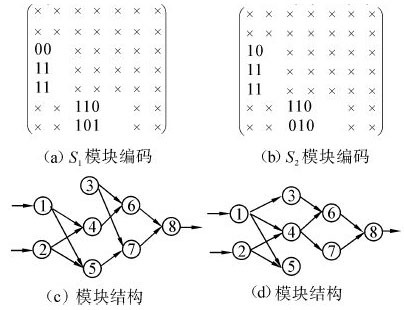图 4 神经网络结构优化 Fig. 4 Neural network structure optimization

1.5 适应度函数及代表个体选择

 $f = \frac{1}{{\frac{1}{T}\sum\limits_{p = 1}^T {\sum\limits_{i = 1}^n {{{\left( {{t_{pi}} - {y_{pi}}} \right)}^2}} } + \alpha }}$ (8)

1.6 算法流程

1) 创建一个初始的神经网络，将待优化神经网络分割成p个模块P1P2，...，Pp

2) 当t=0时，在每个子种群各自搜索空间里，初始化p个进化子种群P1(t)，P2(t)，...，Pp(t)。

3) 对每个进化子种群的个体，选择其代表个体，将其组合成一个合作团体，解码后得到相应的神经网络，依式(8) 计算适应度。

4) 按照笔者所述方法，实施交叉和变异操作，优化神经网络的结构，保留最优个体，形成下一代进化子种群。

5) 判定是否满足算法终止条件(即网络误差足够小或达到进化代数)。若满足，进化结束，并输出优化后的神经网络；否则t=t+1，转到3)。

2 票价格预测仿真股

2.1 数据预处理

 ${{x'}_i} = \frac{{{x_i} - \min x}}{{\max x - \min x}}$ (9)

2.2 性能评价指标

1) 均方误差(MSE)。

 ${\rm{MSE}} = \frac{1}{n}\sum\limits_{i = 1}^n {E_i^2}$ (10)

2) 正确趋势率(PCD)。

 ${\rm{PCD}} = \sum\limits_i {{\rm{pc}}{{\rm{d}}_i}/N}$ (11)

2.3 实验结果分析

2.3.1 HCCGA-BP与FOBS预测结果比较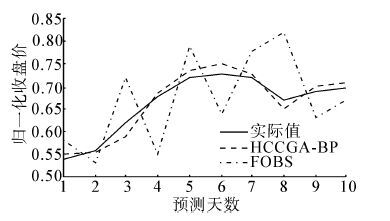图 5 上证综指(000001) 预测收盘价格 Fig. 5 SHCOMP (000001) closing price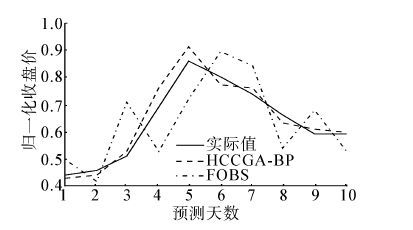图 6 中国石化(600028) 预测收盘价格 Fig. 6 SINOPEC (600028) closing price

 MSE* PCD/% 迭代代数 计算时间/s FOBS 0.007 30 62.1 7 632 86.5 HCCGA-BP 0.000 24 92.1 6 421 78.2 注：归一化

 MSE* PCD/% 迭代代数 计算时间/s FOBS 0.013 46 67.3 6 215 71.2 HCCGA-BP 0.001 10 90.4 5 842 62.3 注：归一化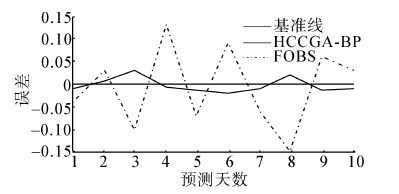图 7 上证综指(000001) 预测均方误差对比 Fig. 7 SHCOMP (000001) error comparison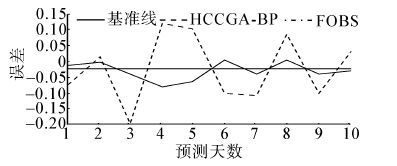图 8 中国石化(600028) 预测均方误差对比 Fig. 8 SINOPEC (600028) error comparison

2.3.2 HCCGA-BP与CCGA-BP、GA-BP预测结果比较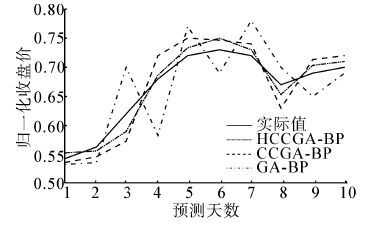图 9 上证综指(000001) 预测收盘价格 Fig. 9 SHCOMP (000001) prediction cosing price图 10 中国石化(600028) 预测收盘价格 Fig. 10 SINOPEC (600028) prediction closing price图 11 上证综指(000001) 预测均方误差对 Fig. 11 SHCOMP (000001)prediction error comparison图 12 中国石化(600028) 预测均方误差对比 Fig. 12 SINOPEC (600028) prediction error comparison

 MSE* PCD/% 迭代代数 计算时间/s GA-BP 0.002 74 69.3 8 153 93.8 CCGA-BP 0.000 86 87.4 7 524 82.6 HCCGA-BP 0.000 24 92.1 6 421 78.2 注：归一化

 MSE* PCD/% 迭代代数 计算时间/s GA-BP 0.006 3 71.9 6 843 74.6 CCGA-BP 0.001 9 86.2 6 216 68.2 HCCGA-BP 0.001 1 90.4 5 842 62.3 注：归一化

3 结束语

  YONG Mingjing, HUA Yingdong. Study on characteristic of fractional master-slave neural network[C]//Fifth International Symposium on Computational Intelligence and Design. Hangzhou, China, 2012: 498-501.  乔俊飞, 张颖. 一种多层前馈神经网络的快速修剪算法[J]. 智能系统学报,2008, 3 (2) : 622 –627. QIAO Junfei, ZHANG Ying. Fast unit pruning algorithm for feed forward neural network design[J]. CAAI Transactions on Intelligent Systems,2008, 3 (2) : 622 –627.  MOOD Y J. Prediction risk and architecture selection for neural networks[C]//Statistics to Neural Networks: Theory and Pattern Recognition Applications, NATO ASI Series F. New York, 1994: 288-290.  LIU Yong. Create stable neural networks by cross-validation[C]//International Joint Conference on IJCNN'06 Neural Networks. Vancouver, Canada, 2006: 3925-3928.  DU Juan, ER Mengjoo. A fast pruning algorithm for an efficient adaptive fuzzy neural network[C]//Eighth IEEE International Conference on Control and Automation. Xiamen, China, 2010: 1030-1035.  乔俊飞, 韩红桂. 前馈神经网络分析与设计. 北京:科学出版社[M]. 2013 : 107 -158.  EUGENE S, MARIA S. A self-configuring genetic programming algorithm with modified uniform crossover[C]//WCCI 2012 IEEE World Congress on Computational Intelligence. Brisbane, Australia, 2012: 1984-1990.  吴鹏. 基于语法引导的遗传编程在神经树中的应用[D]. 济南: 济南大学, 2007: 69-102. WU Peng. The application of genetic programming in the neural tree based on grammar guide[D]. Jinan: University of Jinan, 2007: 69-102.  周明, 孙树栋. 遗传算法原理及应用. 北京:国防工业出版社[M]. 1999 : 1 -26.  巩敦卫, 孙晓燕. 基于合作式协同进化算法的神经网络优化[J]. 中国矿业大学学报,2006, 35 (1) : 114 –119. GONG Dunwei, SUN Xiaoyan. Optimization of neural network based on cooperative co-evolutionary algorithm[J]. Journal of China University of Mining&Technology,2006, 35 (1) : 114 –119.  POTTER M A. The design and analysis of a computational model of cooperative co-evolution [D]. Fairfax: George Mason University, 1997: 21-66.  HETCHT N R. Theory of the back propagation neural network[C]//Proceedings of the International Joint Conference on Neural Networks. New York: IEEE Press, 1989: 593-611.  BLUM E K, LI L K. Approximation theory and feed forward networks[J]. Neural Net,1991, 2 (3) : 511 –515.
DOI: 10.3969/j.issn.1673-4785.201309062

0

#### 文章信息

PU Xingcheng, LIN Yanqin

Hybrid pruning algorithm for the neural network based on significance analysis

CAAI Transactions on Intelligent Systems, 2014, 9(6): 690-697
http://dx.doi.org/10.3969/j.issn.1673-4785.201309062